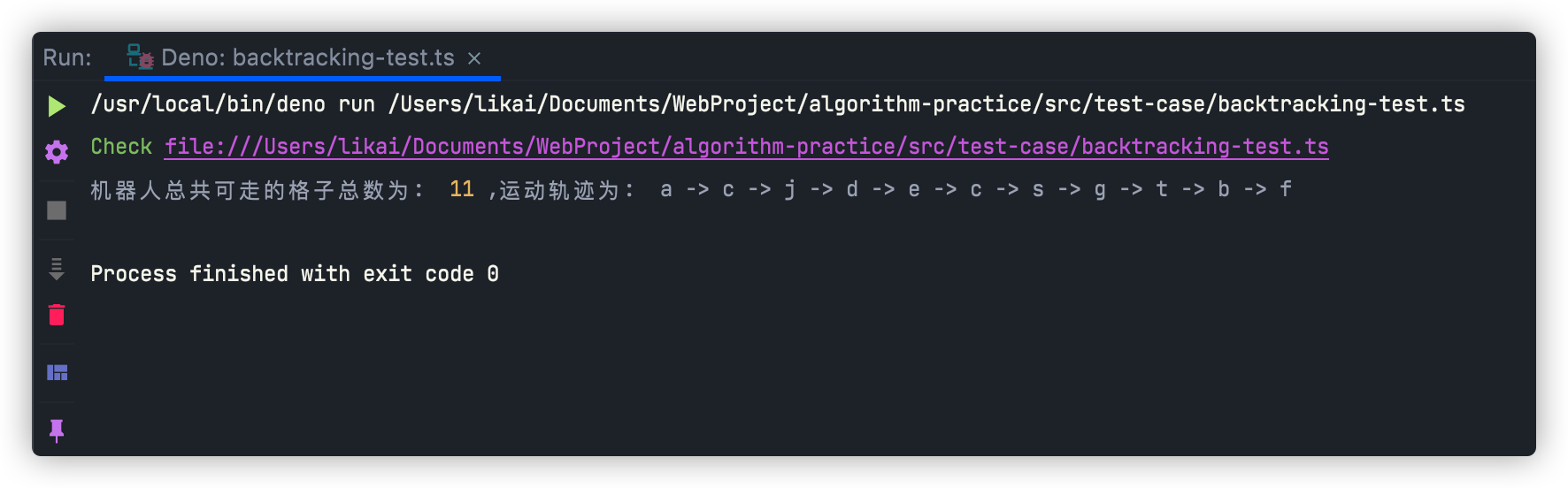• 累计撰写 164 篇文章
• 累计创建 25 个标签
• 累计收到 208 条评论

### 目 录CONTENT# 回溯算法 - 机器人的运动范围2021-08-05 / 0 评论 / 4 点赞 / 294 阅读 / 3,681 字

## 实现思路

### 判断当前格子是否已访问

• 以矩阵的长度为大小创建一个数组
• 遍历创建好的数组，再以矩阵的第0号数组的长度为大小创建数组，赋值给遍历到的每一项。

### 判断格子是否可进入

• 将数字转为字符串，遍历取出每个字符将其转为数字后再相加
• 对数字进行模运算，将其结果相加，再对数字本身进行/10操作，直至数字小于等于0

### 开始移动机器人

• 矩阵的总行数
• 矩阵的总列数
• 即将进入格子的行坐标
• 即将进入格子的列坐标
• 最大活动范围
• 访问标识矩阵
• 路径矩阵

• 待访问格子的行坐标大于矩阵的总行数
• 待访问格子的行坐标小于0
• 待访问格子的列坐标大于矩阵的总列数
• 待访问格子的列坐标小于0
• 当前格子已经被访问
• 当前格子不能进入

## 实现代码

### 格子能否进入函数

  /**
* 判断机器人能否进入目标格子
* @param row 行坐标
* @param col 列坐标
* @param target 临界点
* @private
*/
private checkPath(row: number, col: number, target: number): boolean {
// 两坐标的数位之和必须小于等于临界点
return sumOfDigits(row) + sumOfDigits(col) <= target;
}

// 转字符串实现
export function sumOfDigits(target: number): number {
let finalVal = 0;
const computeVal = target.toString();
for (let i = 0; i < computeVal.length; i++) {
finalVal += Number(computeVal[i]);
}
return finalVal;
}

// 数位之和 - 模运算实现
export function sumOfDigitsForModular(target: number): number {
let finalVal = 0;
while (target > 0) {
finalVal += Math.floor(target % 10);
target /= 10;
}
return finalVal;
}


### 移动机器人函数

  /**
* 开始移动机器人
* @param rows 矩阵总行数
* @param cols 矩阵总列数
* @param row 待进入格子的行坐标
* @param col 待进入格子的列坐标
* @param threshold 最大活动范围
* @param isVisited 访问标识矩阵
* @param matrix 路径矩阵
* @private
*/
private startMoving(
rows: number,
cols: number,
row: number,
col: number,
threshold: number,
isVisited: Array<Array<boolean>>,
matrix: Array<Array<T>>
): number {
// 边界条件判断
if (
row >= rows ||
row < 0 ||
col >= cols ||
col < 0 ||
isVisited[row][col] ||
!this.checkPath(row, col, threshold)
) {
return 0;
}
// 记录当前访问的格子内容
this.path += \${matrix[row][col]} -> ;
// 标识当前格子已被访问
isVisited[row][col] = true;
// 格子访问数量+1
return (
1 +
this.startMoving(rows, cols, row + 1, col, threshold, isVisited, matrix) +
this.startMoving(rows, cols, row, col + 1, threshold, isVisited, matrix) +
this.startMoving(rows, cols, row - 1, col, threshold, isVisited, matrix) +
this.startMoving(rows, cols, row, col - 1, threshold, isVisited, matrix)
);
}


### 主函数

  /**
* 题目：
* 地上有一个m行n列的方格。
* 一个机器人从坐标（0，0）的格子开始移动，
* 它每次可以向左、右、上、下移动一格，但不能进入行坐标和列坐标的数位之和大于k的格子。
* 例如，当k为18时，机器人能够进入方格 （35，37），因为3+5+3+7=18。
* 但它不能进入方格（35，38），因为3+5+3+8=19. 请问该机器人能够到达多少个格子？
* @param matrix 矩阵
* @param threshold 临界点(最大活动范围)
*/
public movingCount(matrix: Array<Array<T>>, threshold = 0): number {
if (threshold < 0 || matrix.length <= 0) {
return 0;
}
// 获取方格的总行数与总列数
const rows = matrix.length;
const cols = matrix.length;
// 创建标记数组，用于标识格子是否被访问
const isVisited: Array<Array<boolean>> = new Array(rows);
for (let i = 0; i < isVisited.length; i++) {
isVisited[i] = new Array(cols);
}
// 从0,0位置开始移动，计算总的移动格子数量
return this.startMoving(rows, cols, 0, 0, threshold, isVisited, matrix);
}


### 编写测试用例

const pathArr = [
["a", "b", "t", "g"],
["c", "f", "c", "s"],
["j", "d", "e", "h"]
];

const backtracking = new Backtracking<string>();
const totalCount = backtracking.movingCount(pathArr, 4);
const path = backtracking.path;
console.log(
"机器人总共可走的格子总数为: ",
totalCount,
",运动轨迹为: ",
path.substr(0, path.length - 3)
);## 写在最后

• 文中如有错误，欢迎在评论区指正，如果这篇文章帮到了你，欢迎点赞和关注😊
• 本文首发于掘金，未经许可禁止转载💌
4